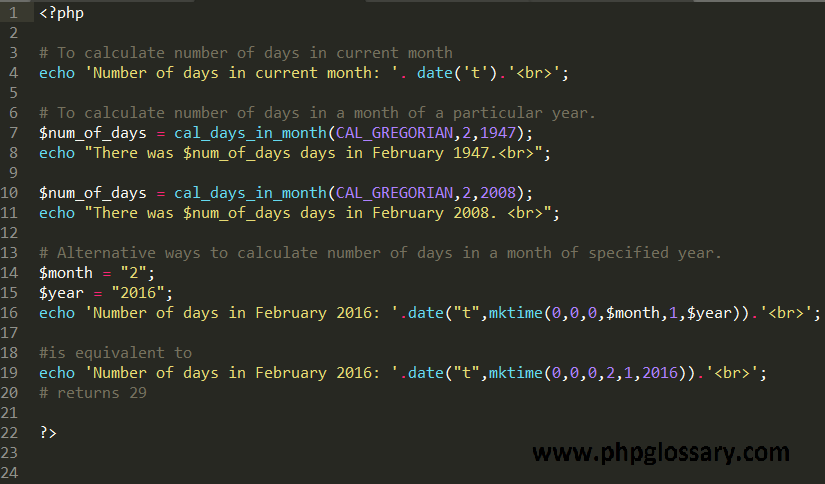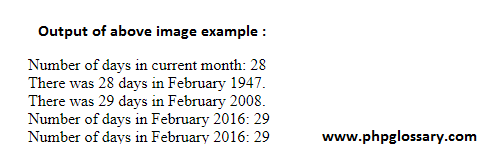# How to calculate number of days in a month of a specified year

To calculate how many days in a particular month of a year PHP provides in build function cal_days_in_month() function returns the number of days in a month for a specified year and calendar.

## The cal_days_in_month() function accepts three parameters :

(1) Calendar: The name of the calendar for days calculation.
(2) Month: Specifies number of a particular month for days calculation.
(3) Year: Specifies year of a particular calendar.

Complete Syntax : cal_days_in_month(calendar,month,year);

The cal_days_in_month() function will return the number of days in the selected month, in the given year and calendar.

Remember if you just want the days in the current month, use the date function:

an alternative way of getting the number of days in a month of the specified year :

To find Number of days in next month
First of all, We will read the present month and present year by using date() function. if we add 1 in present month then we will get next month and by using this trick we can get the number of days in that month like this:

The output is here Number of days in the 03rd month: 31

To find the number of days in the previous month

From the present month, we can subtract 1 to get previous month

\$num_of_days = date(‘t’,mktime(0,0,0,\$month-1,1,\$year)); // to calculate number of days in previous monthThis site uses Akismet to reduce spam. Learn how your comment data is processed.